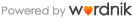# calculate

calculate

### 語源

• calc- : L.calx = lime(石灰); L.calculare = calculate(計算する) < calculus = pebble(（計算用の）小石)

### 意味(英語)

• (verb-transitive) To ascertain by computation; reckon: calculating the area of a circle; calculated their probable time of arrival.
• (verb-transitive) To make an estimate of; evaluate: calculating the team's chances of winning.
• (verb-transitive) To make for a deliberate purpose; design: a sturdy car that is calculated to last for years; a choice that was calculated to please.
• (verb-transitive) Chiefly New England To suppose: "I cal'late she's a right smart cook” ( Dialect Notes).
• (verb-transitive) Chiefly New England To plan, intend, or count on.

### 例文

• I can get around this by defining a body class and calling it, but would like to understand why it is behaving the way it is. getDB (); if ((\$calculate-validateUser (\$userName, \$password) = = "FALSE") or (\$calculate - abduraooft## calcium

calcium

カルシウム

#### 語源

• calc- : L.calx = lime(石灰); L.calculare = calculate(計算する) < calculus = pebble(（計算用の）小石)

## calculable

calculable

#### 語源

• calc- : L.calx = lime(石灰); L.calculare = calculate(計算する) < calculus = pebble(（計算用の）小石)

【広告】## calculated

calculated

#### 語源

• calc- : L.calx = lime(石灰); L.calculare = calculate(計算する) < calculus = pebble(（計算用の）小石)

## calculation

calculation

#### 語源

• calc- : L.calx = lime(石灰); L.calculare = calculate(計算する) < calculus = pebble(（計算用の）小石)

## calculus

calculus

(数学)微積分学、計算法

#### 語源

• calc- : L.calx = lime(石灰); L.calculare = calculate(計算する) < calculus = pebble(（計算用の）小石)